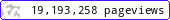•••••MadAsMaths.com :: I.Y.G.B. Practice Papers :: Core Practice Papers 2005 Spec :: C3 Practice Papers

I.Y.G.B. C3 PRACTICE PAPERS

These papers have been written recently and follow the Pearson/Edexcel Syllabus introduced in 2005. The students that have used these papers feel that they are slightly harder than the actual examination papers.

• Papers A to R have standard difficulty with later papers usually more difficult.

• Papers S and T are extremely hard.

• Papers U to Z are hard.

All papers have model solutions and most have guide marking schemes.

The difficulty rating (the second number indicated at the front of each paper) is a very rough UMS converter, so long as the total marks in the paper are 45 or more.

UMS = TOTAL MARKS multiplied by the DIFFICULTY RATING
e.g. total marks = 55
difficulty rating = 1.4033
U.M.S = 77 (approx).

 c3_a.pdfc3_a_marks.pdfc3_a_solutions.pdfc3_b.pdfc3_b_marks.pdfc3_b_solutions.pdfc3_c.pdfc3_c_marks.pdfc3_c_solutions.pdfc3_d.pdfc3_d_marks.pdfc3_d_solutions.pdfc3_e.pdfc3_e_marks.pdfc3_e_solutions.pdfc3_f.pdfc3_f_marks.pdfc3_f_solutions.pdfc3_g.pdfc3_g_marks.pdfc3_g_solutions.pdfc3_h.pdfc3_h_marks.pdfc3_h_solutions.pdfc3_i.pdfc3_i_marks.pdfc3_i_solutions.pdfc3_j.pdfc3_j_marks.pdfc3_j_solutions.pdfc3_k.pdfc3_k_marks.pdfc3_k_solutions.pdfc3_l.pdfc3_l_marks.pdfc3_l_solutions.pdfc3_m.pdfc3_m_marks.pdfc3_m_solutions.pdfc3_n.pdfc3_n_marks.pdfc3_n_solutions.pdfc3_o.pdfc3_o_marks.pdfc3_o_solutions.pdfc3_p.pdfc3_p_marks.pdfc3_p_solutions.pdfc3_q.pdfc3_q_marks.pdfc3_q_solutions.pdfc3_r.pdfc3_r_marks.pdfc3_r_solutions.pdfc3_s.pdfc3_s_marks.pdfc3_s_solutions.pdfc3_t.pdfc3_t_marks.pdfc3_t_solutions.pdfc3_u.pdfc3_u_marks.pdfc3_u_solutions.pdfc3_v.pdfc3_v_marks.pdfc3_v_solutions.pdfc3_w.pdfc3_w_marks.pdfc3_w_solutions.pdfc3_x.pdfc3_x_marks.pdfc3_x_solutions.pdfc3_y.pdfc3_y_marks.pdfc3_y_solutions.pdfc3_z.pdfc3_z_marks.pdfc3_z_solutions.pdf